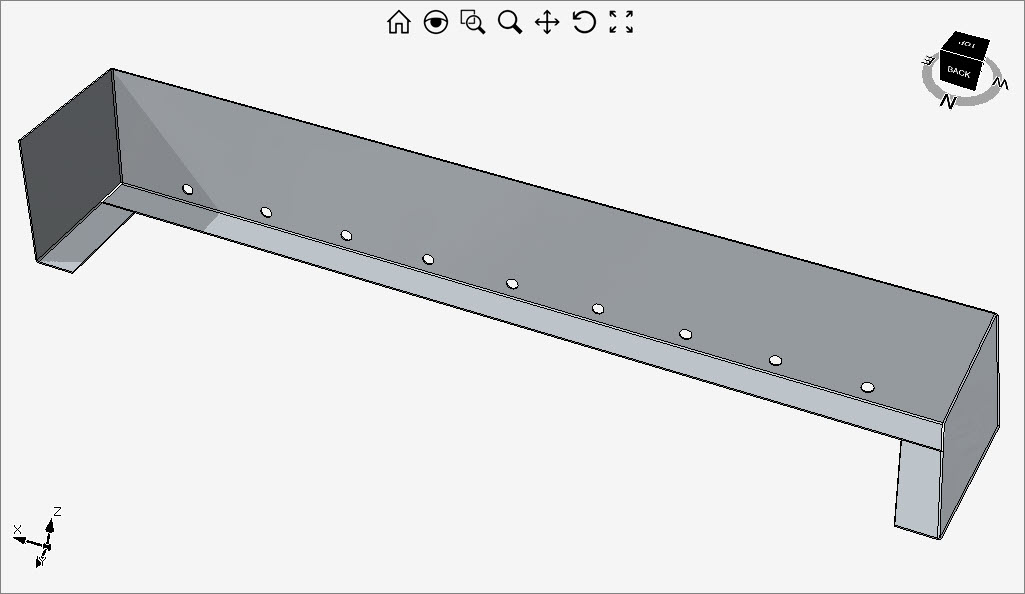# Sheet Metal Cover``````double thickness = 1;

double width = 570 - 2 * radius - 2 * thickness;
double depth = 100 - radius - thickness;
double height = 90 - radius - thickness;
double bend = 25 - radius;

List<ICurve> curveList = new List<ICurve>();
LinearPath lp = new LinearPath(0, 0, width, height);

double margin = 50;
Circle c1 = new Circle(margin, 10, 0, 4);

const int holeCount = 9;
for (int i = 1; i < holeCount; i++)
{
Entity copy = (Entity)c1.Clone();

copy.Translate(i * (width - margin * 2) / (holeCount - 1), 0);

}
devDept.Eyeshot.Entities.Region reg = new devDept.Eyeshot.Entities.Region(curveList);

Brep ext1 = reg.ExtrudeAsBrep(-thickness);

//// long bend
int eIndex = ext1.GetStraightEdgeIndex(new Point3D(0.5, 0, 0));

//// left
eIndex = ext1.GetStraightEdgeIndex(new Point3D(0, thickness / 2, 0));

//// face split
Plane splitPln = Plane.XY;
splitPln.Translate(0, 0, radius + bend + thickness);

ext1.Rebuild();

int fIndex = ext1.GetPlanarFaceIndex(Plane.XZ, new Point3D(-radius - thickness / 2, 0, +radius + thickness / 2));
ext1.SubdivideBy(fIndex, splitPln);

eIndex = ext1.GetStraightEdgeIndex(new Point3D(-radius, 0, bend + radius + thickness + thickness / 2));

//// right
eIndex = ext1.GetStraightEdgeIndex(new Point3D(width, thickness / 2, 0));

//// face split
ext1.Rebuild();

fIndex = ext1.GetPlanarFaceIndex(Plane.XZ, new Point3D(width + radius + thickness / 2, 0, bend + radius + thickness / 2));
ext1.SubdivideBy(fIndex, splitPln);

eIndex = ext1.GetStraightEdgeIndex(new Point3D(width + radius, 0, bend + radius + thickness * 2));

``````
1 out of 1 found this helpful
Have more questions? Submit a request

• This is really great work to see. Very happy with this.

Also, I see a related "ExtrudeFace()" that is useful for "push/pull" ability of planar faces. Again, great work.

A related question... "AddFlange" ability seems similar to inserting a 3d fillet in place of an edge (perhaps I am overlooking many details). Just checking if this is still a planned improvement.

• Hi James,